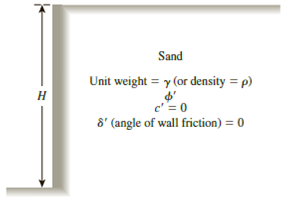Chapter 13, Problem 13.3PPrinciples of Geotechnical Enginee...

9th Edition
Braja M. Das + 1 other
ISBN: 9781305970939

Solutions

Chapter
SectionPrinciples of Geotechnical Enginee...

9th Edition
Braja M. Das + 1 other
ISBN: 9781305970939
Textbook Problem

13.1 through 13.4 Figure 13.39 shows a retaining wall that is restrained from yielding. For each problem, determine the magnitude of the lateral earth force per unit length of the wall. Also, find the location of the resultant, z ¯ , measured from the bottom of the wall.Figure 13.39

To determine

Find the magnitude of the lateral earth forces Po per unit length of the wall and find the location z¯ of the resultant measured from the bottom of the wall.

Explanation

Given information:

The height (H) of the retaining wall is 11 m.

The soil friction angle ϕ is 28°.

The angle of wall friction δ is 0.

The unit weight γ of the sand is 17.9kN/m3

The over-consolidation ratio (OCR) is 1.3.

Calculation:

Calculate the earth pressure coefficient (Ko) using the relation.

Ko=(1sinϕ)(OCR)sinϕ

Substitute 28° for ϕ and 1.3 for OCR.

Ko=(1sin28°)(1.3)sin28°=0.53053×1.13108=0.60

Calculate the total force per unit length (Po) using the relation.

Po=12KoγH2

Substitute 0

Still sussing out bartleby?

Check out a sample textbook solution.

See a sample solution

The Solution to Your Study Problems

Bartleby provides explanations to thousands of textbook problems written by our experts, many with advanced degrees!

Get Started

How has computer security evolved into modern information security?

Principles of Information Security (MindTap Course List)

Under the CAFE standards, for what are vehicles tested?

Automotive Technology: A Systems Approach (MindTap Course List)

What is the EPEAT? How is it used?

Principles of Information Systems (MindTap Course List)

Discuss the importance of data models.

Database Systems: Design, Implementation, & Management

Repeat the previous problem using sx= 12 MPa.

Mechanics of Materials (MindTap Course List)

How would you balance DFDs?

Systems Analysis and Design (Shelly Cashman Series) (MindTap Course List)

What does the kernel do?

Enhanced Discovering Computers 2017 (Shelly Cashman Series) (MindTap Course List)

Why must a mushroomed chisel or hammer be reground?

Welding: Principles and Applications (MindTap Course List)

What three characteristics about TCP distinguish it from UDP?

Network+ Guide to Networks (MindTap Course List)